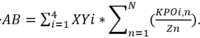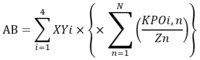# Basic Summation

#### morgenblatter

##### New member
I’m having difficult knowing what questions I need to ask the author to resolve the following formula which appears as follows. There are curly braces around the second sigma group, but I do not see that as having any mathematical significance. XYi is a value obtained from a table which I don’t have at moment. I’m assuming that the “i” is the same as the index in the first Summation. The uppercase N in the second part is currently undefined unless there is some convention that assigns it a value. KPO and Z in the second part are defined and I believe the “i” and the “n” in the second part both relate to the indices so that in the first iteration, they would both equal 1.
Can someone confirm that I need to ask for the value of “N” and can anyone rewrite the formula in simple algebraic format so that I can express AB in an Excel cell?

#### Subhotosh Khan

##### Super Moderator
Staff member
I’m having difficult knowing what questions I need to ask the author to resolve the following formula which appears as follows View attachment 20730 . There are curly braces around the second sigma group, but I do not see that as having any mathematical significance. XYi is a value obtained from a table which I don’t have at moment. I’m assuming that the “i” is the same as the index in the first Summation. The uppercase N in the second part is currently undefined unless there is some convention that assigns it a value. KPO and Z in the second part are defined and I believe the “i” and the “n” in the second part both relate to the indices so that in the first iteration, they would both equal 1.
Can someone confirm that I need to ask for the value of “N” and can anyone rewrite the formula in simple algebraic format so that I can express AB in an Excel cell?
Can you paste an image of the given equation from the given reference?

#### morgenblatter

##### New member
Hi Subhotosh,
Thanks for the reply. Here is the originalBy the way, I have a suspicion that the lowercase i's and n's may not be related to the indices, but if this were the case and the expressions were in fact constants, the structure seems an overly complicated way of expressing the formula. On the other hand, if they are related to the indices, they seem to be lacking a mathematical operator.

#### Jomo

##### Elite Member
Not sure why there are two multiplication symbols next to one another.

I am not sure what you are having trouble with.

Can you please write out what you get when i = 1? That way we can see where you are having trouble. Thanks.

#### morgenblatter

##### New member
Hi Jomo,
Thanks for your interest, but I have no idea how to get any values out of the formula. It has been given to me, and it seems to me either that the "i's" and "n's" within the formula require an operator to determine how they are to be used, or they are not related to the indices, in which case, as far as the formula is concerned they are constants. If so, then it seems to be a wonderfully elaborate way of writing AB = 4*XYi*N*KPOi/Zn. Unfortunately, I currently do not have a clear definition of XYi, KPOi or Zn and the Uppercase "N" is a complete mystery. As for my query, I would like confirmation that my suppositions are correct and that I'm not missing something. Please bear in mind that my highest achievement in mathematics was a gold star for "sums" in a mixed infants class and I'm wondering if that's why I have a problem with the formula or whether perhaps the author of the formula never managed to reach this level. By the way, I'm heartened to learn that you also had trouble with the double multiplication.